## Problem Description

Given n non-negative integers representing an elevation map where the width of each bar is 1, compute how much water it is able to trap after raining.

For example,
Given [0,1,0,2,1,0,1,3,2,1,2,1], return 6.

problem link:
https://leetcode.com/problems/trapping-rain-water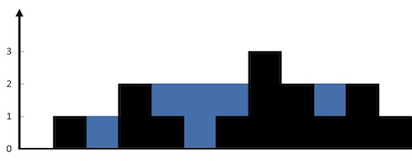## Consumer 拉取消息的实现

### Consumer 拉取消息的数量

org.apache.kafka.clients.consumer.internals.Fetcher#fetchedRecords 可以看到 maxPollRecords(max.poll.records 配置) 变量限制了每次 poll 的消息条数，不管 consumer 对应多少个 partition，从所有 partition 拉取到的消息条数总和不会超过 maxPollRecords

org.apache.kafka.clients.consumer.internals.Fetcher#sendFetches 可以看到 fetchSize(max.partition.fetch.bytes 配置) 用于每次创建 FetchRequest 时的 org.apache.kafka.common.requests.FetchRequest.PartitionData 的参数设置。fetchSize限制了 consumer 每次从每个 partition 拉取的数据量。

The maximum amount of data per-partition the server will return. Records are fetched in batches by the consumer. If the first record batch in the first non-empty partition of the fetch is larger than this limit, the batch will still be returned to ensure that the consumer can make progress. The maximum record batch size accepted by the broker is defined via message.max.bytes (broker config) or max.message.bytes (topic config). See “ + FETCH_MAX_BYTES_CONFIG + “ for limiting the consumer request size.

## Consumer 的心跳机制

org.apache.kafka.clients.consumer.internals.AbstractCoordinat 中启动 HeartbeatThread 线程来定时发送心跳和检查 consumer 的状态。

### sessionTimeout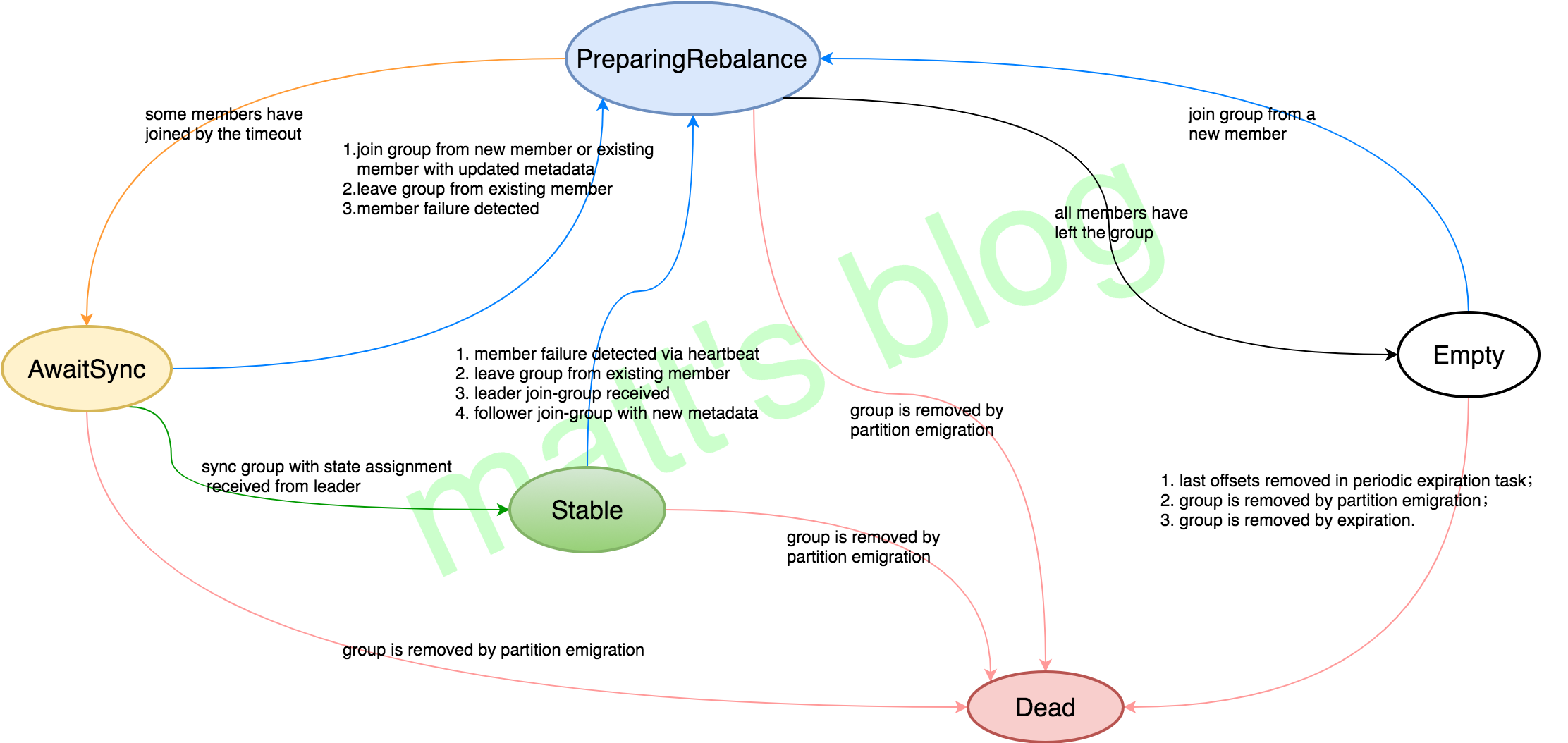### pollTimeout

#### Rebalance Generation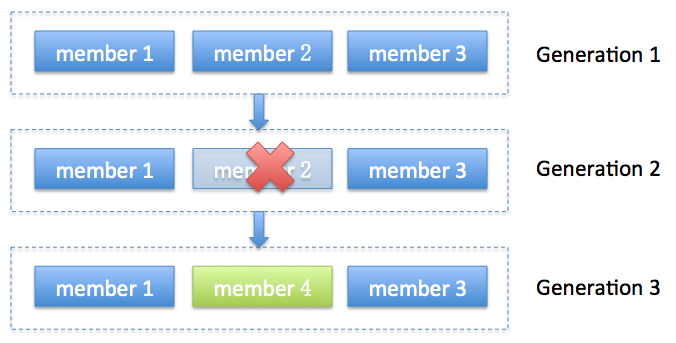## partition 的数量设置

• 一个 partition 只能被 Consumer Group 中的一个 consumer 消费，因此，为了提高并发量，可以提高 partition 的数量，但是这会造成 replica 副本拷贝的网络请求增加，故障恢复时的耗时增加。因为 kafka 使用 batch pull 的方式，所以单个线程的消费速率还是有保障的。并且 partition 数量过多，zk 维护 ISR 列表负载较重。

• partiton 数量最好是 consumer 数目的整数倍，比如取 24， consumer 数目的设置就会灵活很多。

• consumer 消费消息时不时严格有序的。当从多个 partition 读数据时，kafka 只保证在一个 partition 上数据是有序的，多个 partition 的消息消费很可能就不是严格有序的了。

## 参数设置

### session.timeout.ms

consumer session 过期时间。如果超时时间范围内，没有收到消费者的心跳，broker 会把这个消费者置为失效，并触发消费者负载均衡。因为只有在调用 poll 方法时才会发送心跳，更大的 session 超时时间允许消费者在 poll 循环周期内处理消息内容，尽管这会有花费更长时间检测失效的代价。如果想控制消费者处理消息的时间，

### max.poll.interval.ms

This config sets the maximum delay between client calls to poll().

When the timeout expires, the consumer will stop sending heartbeats and send an explicit LeaveGroup request.

As soon as the consumer resumes processing with another call to poll(), the consumer will rejoin the group.

By increasing the interval between expected polls, you can give the consumer more time to handle a batch of records returned frompoll(long). The drawback is that increasing this value may delay a group rebalance since the consumer will only join the rebalance inside the call to poll. You can use this setting to bound the time to finish a rebalance, but you risk slower progress if the consumer cannot actually call poll often enough.

### max.poll.records

Use this setting to limit the total records returned from a single call to poll. This can make it easier to predict the maximum that must be handled within each poll interval. By tuning this value, you may be able to reduce the poll interval, which will reduce the impact of group rebalancing.

0.11.0 Kafka 的默认配置是

• max.poll.interval.ms=5min
• max.poll.records=500

## Kafka Javadoc - Detecting Consumer Failures

After subscribing to a set of topics, the consumer will automatically join the group when poll(long) is invoked. The poll API is designed to ensure consumer liveness. As long as you continue to call poll, the consumer will stay in the group and continue to receive messages from the partitions it was assigned. Underneath the covers, the consumer sends periodic heartbeats to the server. If the consumer crashes or is unable to send heartbeats for a duration of session.timeout.ms, then the consumer will be considered dead and its partitions will be reassigned.
It is also possible that the consumer could encounter a “livelock” situation where it is continuing to send heartbeats, but no progress is being made. To prevent the consumer from holding onto its partitions indefinitely in this case, we provide a liveness detection mechanism using the max.poll.interval.ms setting. Basically if you don’t call poll at least as frequently as the configured max interval, then the client will proactively leave the group so that another consumer can take over its partitions. When this happens, you may see an offset commit failure (as indicated by a CommitFailedException thrown from a call to commitSync()). This is a safety mechanism which guarantees that only active members of the group are able to commit offsets. So to stay in the group, you must continue to call poll.

## 运行环境说明

kafka 版本号为 0.11.0

Kafka Consumer 的参数配置如下：

## Consumer 卡顿现象

### Consumer 卡顿时的日志

2017/11/09 19:35:29:DEBUG pool-16-thread-13 org.apache.kafka.clients.consumer.internals.AbstractCoordinator - Attempt to heartbeat failed for group myConsumerGroup since it is rebalancing.
2017/11/09 19:35:29:INFO pool-16-thread-13 org.apache.kafka.clients.consumer.internals.ConsumerCoordinator - Revoking previously assigned partitions [my_topic-18] for group myConsumerGroup
2017/11/09 19:35:29:INFO pool-16-thread-13 org.springframework.kafka.listener.ConcurrentMessageListenerContainer - partitions revoked: [my_topic-18]

2017/11/09 19:35:29:INFO pool-16-thread-13 org.apache.kafka.clients.consumer.internals.AbstractCoordinator - (Re-)joining group myConsumerGroup

## 卡顿原因分析

### 卡顿原因：Consumer 在 Region Group

ConsumerCoordinator#poll 中判断 needRejoin() 为 true 时会调用 ensureActiveGroup() 函数，如下：

# kafka metadata 什么时候变化？？？？

## Consumer Leave Group 原因：pollTimeoutExpired

org.apache.kafka.clients.consumer.internals.AbstractCoordinator.HeartbeatThread#run 中调用了 maybeLeaveGroup() 函数，其实现如下：

### 根本原因：pollTimeoutExpired

pollTimeoutExpired 的原因是两次 poll 的时间间隔超过了设置的 maxPollInterval 值。

## 解决方案

• max.poll.records：100 (默认值 500)
• max.poll.interval.ms：600000 (默认值 300000，也就是5分钟)

## 后续

• 对于这两个参数值的设定， 是 max.poll.records 越小越好，max.poll.interval.ms 越大越好吗？
• 已经设置过的 session.timeout.msheartbeat.interval.ms难道没用吗？为什么有这么多超时参数的设置啊？
• 已经设置过的 max.partition.fetch.bytes 没用吗？为什么还要设置 max.poll.records 啊？
• 整体上还需要调哪些参数才可以让 consumer 运行正常，或者是性能达到最大呢？

## 1. 指数分布族(Exponential Family)

#### 指数分布族的定义

$$P(y;\eta) = b(y)exp(\eta^TT(y)-a(\eta))$$

• $\eta$: 自然参数，通常是一个实数
• T(y): 充分统计量，通常，T(y)=y，实际上是一个概率分布的充分统计量（统计学知识）
• a($\eta$) 被称为 log partition function

#### 指数分布族以及它们的特征

• 正态分布（高斯分布）——总体噪音（由中心极限定理得）
• 伯努利分布——逻辑回归（对01问题建模）
• 多项式分布——K种结果的事情进行建模
• 泊松分布——对计数过程进行建模（一个样本中放射性衰变的数目，网站的访客数目，商店的顾客数目）
• 伽马分布，指数分布——正数的分布，对间隔进行建模（在公交车站等车的时间）
• β分布，Dirichlet分布——对小数进行分布，对概率分布进行建模
• Wishart分布——协方差的分布

## 2. 指数分布簇推导

### 2.1 伯努利分布的推导

$$P(y;\phi)=\phi^y(1-\phi)^y$$
$$=exp(log(\phi^y(1-\phi)^y))=exp(ylog(\phi)+ (1-y)log(1-\phi))$$
$$=exp(log(\frac\phi{1-\phi})y + log(1-\phi))$$

$T(y) = y$
$b(y) = 1$
$\eta = log(\frac\phi{1-\phi}) => \phi=\frac1{1+e^{-n}}$
$a(\eta) = -log(1-\phi) = log(1+e^n)$

### 2.2 高斯分布的推导

$$P(y;\mu)=\frac{1}{\sqrt{2\pi}}exp(-\frac12(y-\mu)^2)$$
$$=\frac{1}{\sqrt{2\pi}}exp(-\frac{1}{2}y^2) * exp({\mu}y-\frac{1}{2}\mu^2)$$

$T(y) = y$
$b(y) = \frac{1}{\sqrt{2\pi}}exp(-\frac12y^2)$
$\eta = \mu$
$a(\eta) = \frac{{\mu}^2}2 = \frac{{\eta}^2}2$

## 3. 广义线性模型(Generalized Linear Model)

1. $y | x;θ \sim ExponentialFamily(\eta)$ y的条件概率属于指数分布族;
2. 给定 x 广义线性模型的目标是求解 T(y) | x， 不过由于 很多情况下 T(y) = y 所以我们的目标变成了 y | x , 也即 我们希望拟合函数为 h(x) = E[y|x] (这个条件在线性回归和逻辑回归中都满足， 例如在逻辑回归中 $hθ(x) = p(y = 1|x;\theta) = 0 \cdot p(y = 0|x; \theta) + 1 \cdot p(y = 1|x; \theta) = E[y|x;\theta])$
3. 自然参数 $\eta$ 与 x 是线性关系：$\eta=\theta^Tx$ ($\eta 为向量时 \eta_{i} = \theta_{i}^Tx$)

## 4. 多分类算法(Softmax Regression)

y有多个可能的分类：{1, 2, …, k}

=======具体的公式略=======

## 1. 逻辑回归

$$h_θ(x) = g(θ^{T}x)=\frac1{1+e^{-θ^{T}x}}$$

$$g(z)=\frac1{1+e^{-z}}$$；

g(z) 被称为 logistic function 或 sigmoid function，其二维坐标下的曲线为: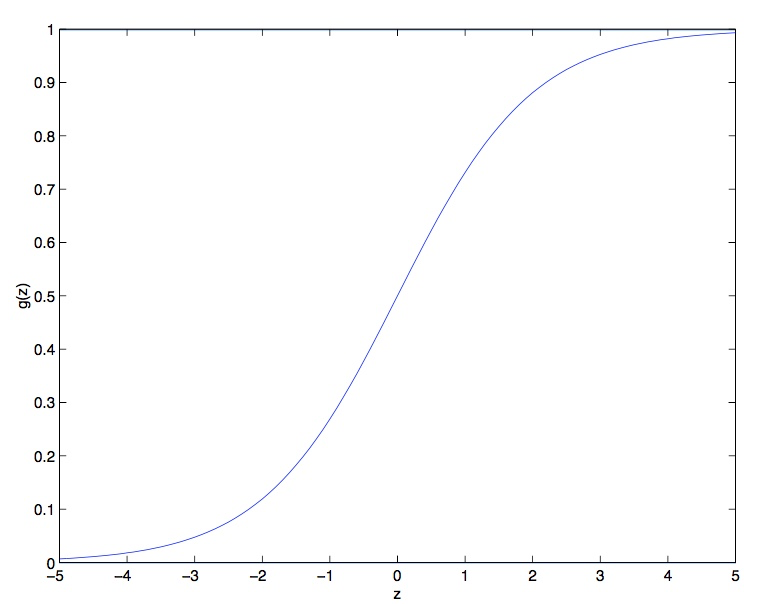g(z) 的导数我们可以先进行推导:
$$g’(z)=\frac{d}{dz}\frac{1}{1+e^{-z}}= \frac{1}{(1+e^{-z})^2}(e^{-z})$$
$$= \frac{1}{1+e^{-z}}*(1 - \frac{1}{1+e^{-z}})= g(z)(1-g(z))$$

## 2. 梯度上升法求解逻辑回归

$P(y=1|x;θ)=h_θ(x)$，代表对于给定的 θ，y 取值为 1 的概率。
$P(y=0|x;θ)=1-h_θ(x)$，代表对于给定的 θ，y 取值为 0 的概率。

$$P(y|x;θ)=(h_θ(x))^y(1 − h_θ(x))^{(1−y)}$$

$$L(θ)=P(\overrightarrow{y}|X;θ)$$
$$= \prod^m_{i=1}P(y^i|x^i;θ)$$
$$= \prod^m_{i=1}{(h_θ(x^{(i)}))^{y^{(i)}}(1 − h_θ(x^{(i)}))^{(1−y^{(i)})}}$$

$l(θ) = logL(θ) = \sum_{m}^{i=1}{y^{(i)}}log{h(x^{(i)}) + {(1−y^{(i)})}log(1 − h(x^{(i)}))}$

$$θ:= θ + \alpha\Deltaθl(θ)$$

$$\frac\partial{\partial\theta_j}l(\theta)$$
$$= (y\frac1{g(\theta^Tx)} - (1-y)\frac1{1-g(\theta^Tx)})\frac\partial{\partial\theta_j}g(\theta^Tx)$$
$$= (y\frac1{g(\theta^Tx)} - (1-y)\frac1{1-g(\theta^Tx)}) g(\theta^Tx)(1-g(\theta^Tx))\frac\partial{\partial\theta_j}\theta^Tx$$
$$= ({y(1-g(\theta^Tx))-(1-y)g(\theta^Tx)})x_j$$
$$= (y - h_{\theta}(x))x_j$$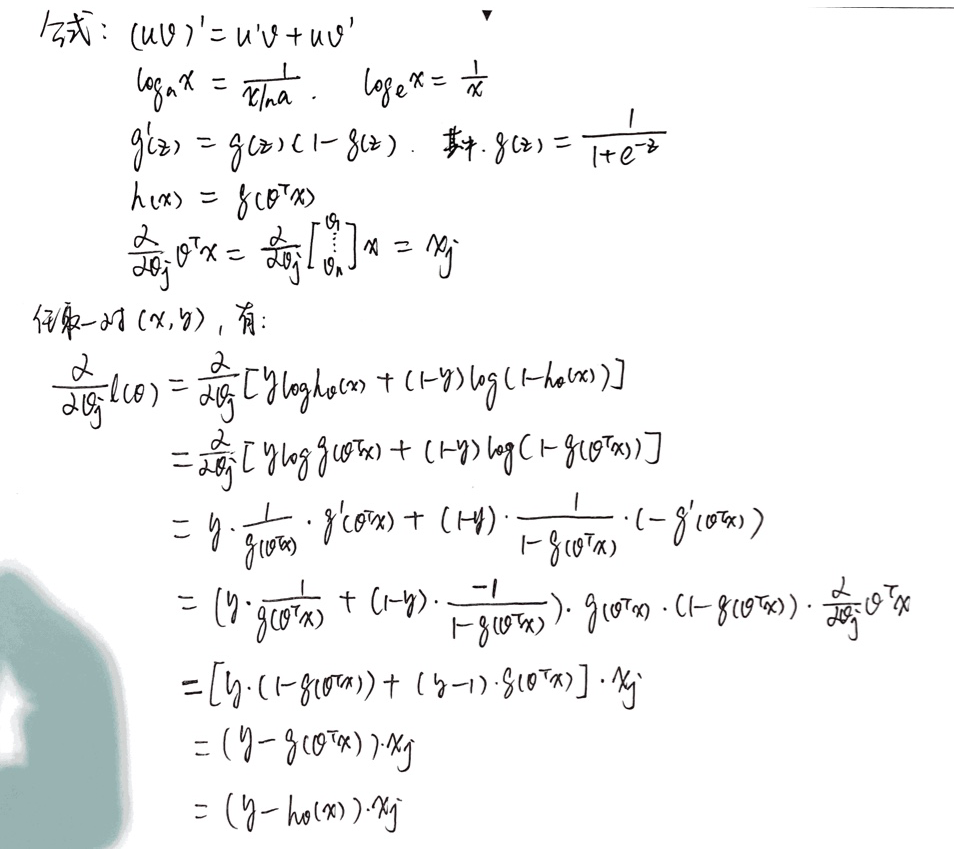$$θ_j:=θ_j + \alpha\sum_{i=1}^{m}(y^{(i)} - h_{\theta}(x^{(i))})x_j^{(i)}$$

$$θ_j = θ_j - α \frac1m * \sum_{i=1}^{m}{(h_θ(x^{(i)}) - y^{(i)})}*x_j^{(i)}$$

## 3. 牛顿迭代法求解逻辑回归

### 3.1 牛顿迭代法简述

$$y-f(x_n) = f’(x_n)(x-x_n)$$

### 3.2 迭代过程

$$\theta^{(t+1)} = \theta^{(t)} - \frac{f(\theta^{(t)})}{f’(\theta^{(t)})}$$

#### 从泰勒展开到牛顿迭代

$$f(x+\Delta x) = f(x)+f’(x)\Delta x+ \frac1{2}f’’(x)\Delta x^2$$

$$f’(x)+ \frac1{2}f’’(x)\Delta x = 0$$

$$\Delta x = -\frac{f’(x)}{f’’(x)}$$

>

1. 将原函数y=f(x)，对x求一次导数，得到dy/dx；
2. 令dy/dx = 0，解得一次导函数的零点；
3. 将原函数对x求二次导函数；
4. 将解得的零点坐标的x值代入二次导函数，
如果是正值，零点所在位置，就是极小值点，再将该x值代入原函数，得到极小值；
如果是值值，零点所在位置，就是极大值点，再将该x值代入原函数，得到极大值；
如果是0，零点所在位置，既不是极小值点，也不是极大值点，是拐点。

$$\theta^{(t+1)} = \theta^{(t)} - \frac{l’(\theta^{(t)})}{l’’(\theta^{(t)})}$$

### 3.3 多维向量的牛顿迭代

$$\theta := \theta - H^{-1} \nabla l(\theta)$$

$\nabla l(\theta)$ 是对 $l(\theta)$ 求导的值。

H 是一个 n*n 的矩阵，n 是特征数量，元素的计算公式为：
$$H_ij= \frac{\partial^2{l({\theta)}}}{\partial{\theta_i}\partial{\theta_j}}$$

## 1. 欠拟合与过拟合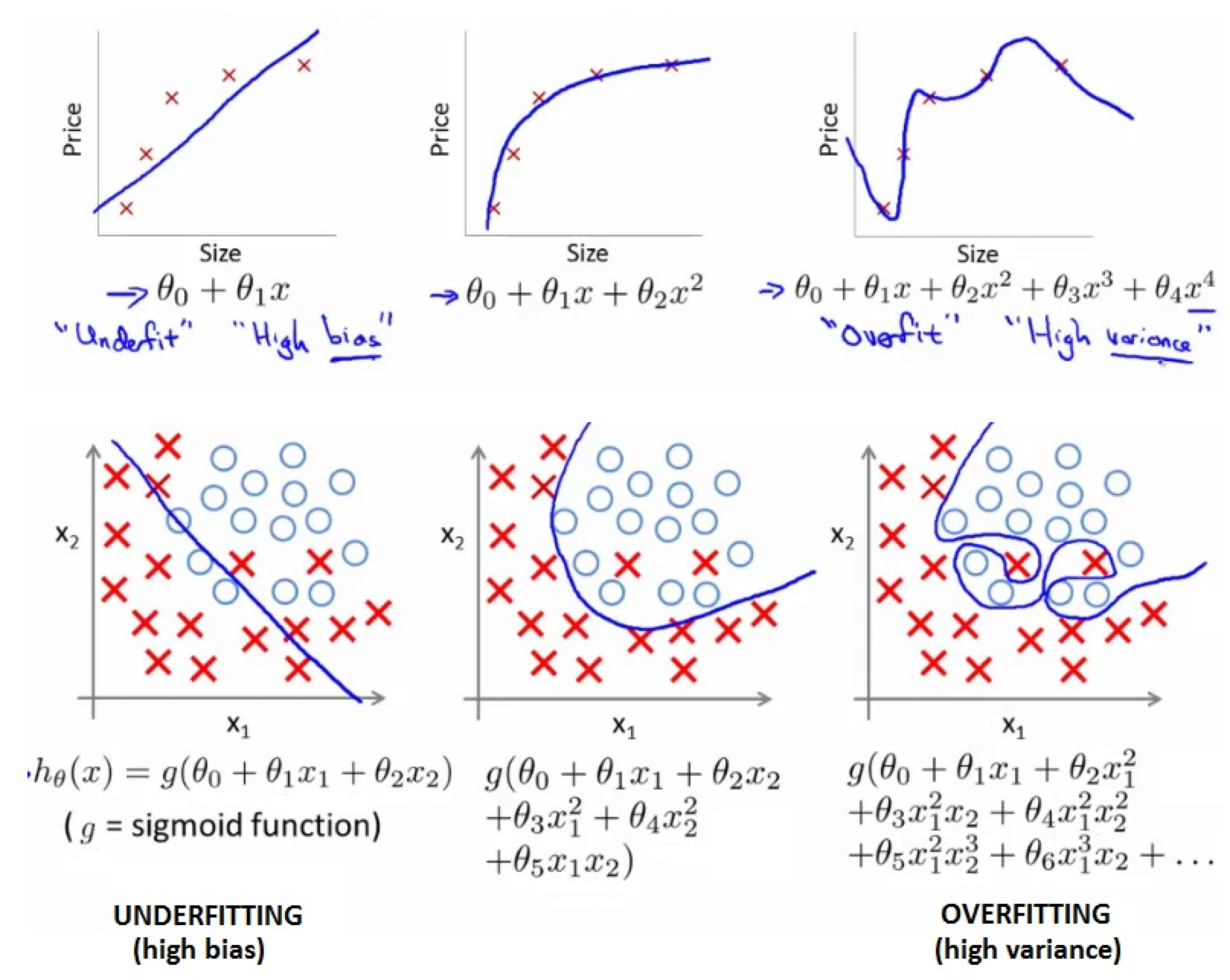## 2. 线性模型的概率解释

### 推导过程

$$y^{(i)}=\theta^Tx^{(i)} + ε^{(i)}$$

Andrew Ng 讲到在大多数情况下，线性回归的误差值如果综合来看，就是符合高斯分布的。并且根据中心极限定律，正态分布确实是对误差项分布的合理猜想。

$$P(y^{(i)}|x^{(i)}; θ) = \frac{1}{\sqrt{2\pi}\sigma}exp(- \frac{(y^{(i)}-\theta^Tx^{(i)})^2}{2\sigma^2})$$

$P(y^{(i)}|x^{(i)}; θ)$ 表示：在 θ 为给定的参数的情况下，概率 $y^{(i)}$ 以 $x^{(i)}$ 为随机变量的概率分布，注意 θ 不是随机变量。

$$L(θ)=L(θ;X,Y)=p(Y|X;θ) = \prod_{i=1}^{m}\frac{1}{\sqrt{2\pi}\sigma}exp(- \frac{(y^{(i)}-\theta^Tx^{(i)})^2}{2\sigma^2})$$

$$l(\theta)=logL(\theta) = log\prod_{i=1}^{m}\frac{1}{\sqrt{2\pi}\sigma}exp(- \frac{(y^{(i)}-\theta^Tx^{(i)})^2}{2\sigma^2})$$
$$= m\sum_{i=1}^{m}log\frac{1}{\sqrt{2\pi}\sigma} - \frac1{2\sigma^2}\sum_{i=1}^{m}(y^{(i)}-\theta^Tx^{(i)})^2$$

# Reference

## 1. 多变量的线性回归

n: 特征(features) 数量
m: 训练集数量
$x^{(i)}$:

• 表示一条训练数据的向量
• i is an index into the training set
• So
• x is an n-dimensional feature vector
• $x^{(3)}$ is, for example, the 3rd training data

$x^{(j)}_i$: The value of feature j in the ith training example

$$h_θ(x) = θ_0 + θ_1x_1 + θ_2x_2 + θ_3x_3 + θ_4x_4$$

For convenience of notation, $x_0$ = 1, 所以最后的特征向量的维度是 n+1，从 0 开始，记为”X”，

$$h_θ(x)=θ^TX$$
$θ^T$: [1 * (n+1)] matrix

### Cost Function

$$J(θ_0, θ_1, …,θ_n) = \frac1{2m}\sum_{i=1}^{m}{(h_θ(x^{(i)}) - y^{(i)})^2}$$

### Gradient descent

Repeat {
$$θ_j = θ_j - α\frac\partial{\partial J(θ_0, θ_1, …,θ_n)}$$
}

every iterator

• θj = θj - learning rate (α) times the partial derivative of J(θ) with respect to θJ(…)
• We do this through a simultaneous update of every θj value

$$\frac\partial{\partial J(θ_0, θ_1, …,θ_n)}$$
$$= \frac1m * \sum_{i=1}^{m}{(h_θ(x^{(i)}) - y^{(i)})}*x_j^{(i)}$$

## 2. Gradient Decent in practice

### Learning Rate α

• working correctly: If gradient descent is working then J(θ) should decrease after every iteration
• convergence: 收敛是指每经过一次迭代，J(θ)的值都变化甚小。
• choose α
1. When to use a smaller α
• If J(θ) is increasing, see below picture
• If J(θ) looks like a series of waves, decreasing and increasing again
• But if α is too small then rate is too slow
2. Try a range of α values
• Plot J(θ) vs number of iterations for each version of alpha
• Go for roughly threefold increases: 0.001, 0.003, 0.01, 0.03. 0.1, 0.3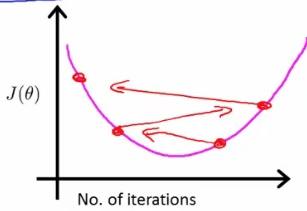## 2.2 Features and polynomial regression

### Polynomial regression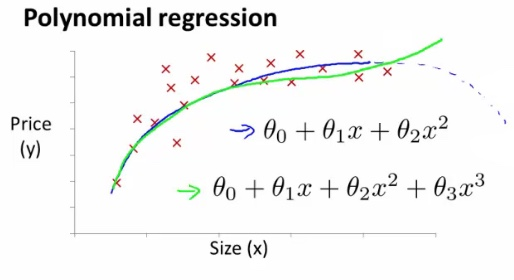## 3. Normal equation 求解多变量线性回归

### 3.1 Normal equation

$$J(θ_0, θ_1, …,θ_n) = \frac1{2m}\sum_{i=1}^{m}{(h_θ(x^{(i)}) - y^{(i)})^2}$$

$$\frac\partial{\partial θ_j}J(θ_0, θ_1, …,θ_n) = … = 0$$，其中 j = 0,1,2,…,n

$$θ = (X^TX)^{-1}X^Ty$$

## 4. Gradient descent Vs Normal equation

### Gradient descent

• Need to chose learning rate
• Needs many iterations - could make it slower
• Works well even when n is massive (millions)
• Better suited to big data
• What is a big n though: 100 or even a 1000 is still (relativity) small, If n is 10000 then look at using gradient descent
• 适用于线性回归会逻辑回归

### Normal equation

• No need to chose a learning rate
• No need to iterate, check for convergence etc.
• Normal equation needs to compute $(X^TX)^{-1}$
• This is the inverse of an n x n matrix
• With most implementations computing a matrix inverse grows by O(n3), So not great
• Slow of n is large, Can be much slower
• 仅适用于线性回归

## 5. 局部加权线性回归

$$J(\theta) = \sum_{i=1}^{m} w^{(i)}( y^{(i)}-\theta^Tx^{(i)} )^2$$

$$w^{(i)} = exp (-\frac{(x^{(i)}-x)^2}{\tau^2})$$

$w^{(i)}$的形式跟正态分布很相似，但二者没有任何关系，仅仅只是便于计算。可以发现，$x^{(j)}$ 离 $x^{(i)}$ 非常近时，${w^{(i)}_j}$ 的值接近于1，此时 j 点的贡献很大，当 $x^{(j)}$ 离 $x^{(i)}$ 非常远时，${w^{(i)}_j}$ 的值接近于 0，此时 j 点的贡献很小。

$\tau^2$ 是波长函数(bandwidth)， 控制权重随距离下降的速度，τ 越小则 x 离 $x^{(i)}$ 越远时 $w^{(i)}$ 的值下降的越快。

• 每次对一个点的预测都需要整个数据集的参与，样本量大且需要多点预测时效率低。提高效率的方法参考 Andrew More’s KD Tree
• 不可外推，对样本所包含的区域外的点进行预测时效果不好，事实上这也是一般线性回归的弱点

## Reference

http://www.holehouse.org/mlclass/04_Linear_Regression_with_multiple_variables.html

## 2. 单变量线性回归

$h_{\theta}$(x) = $\theta_{0}$ + $\theta_{1}$

## 3. Cost Function

$$\frac1{2m}\sum_{i=1}^{m}{(h_{\theta}(x^{(i)}) - y^{(i)})^2}$$ 最小的 $\theta$ 值。

$$J(\theta_0, \theta_1) = \frac1{2m}\sum_{i=1}^{m}{(h_{\theta}(x^{(i)}) - y^{(i)})^2}$$

#### Cost Function 的作用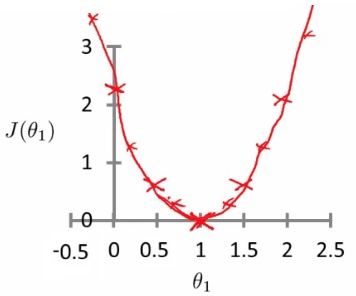$$J(\theta_1)= \frac1{2m}\sum_{i=1}^{m}{(\theta_1x^{(i)} - y^{(i)})^2}$$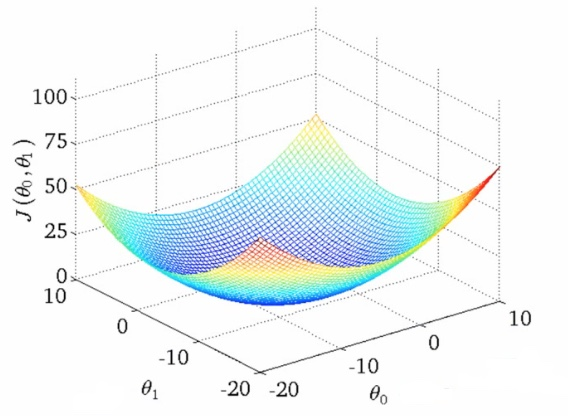## 4. 梯度下降法

• Start with initial guesses
• Start at 0,0 (or any other value)
• Keeping changing $\theta_0$ and $\theta_1$ a little bit to try and reduce J($\theta_0$, $\theta_1$)
• Each time you change the parameters, you select the gradient which reduces J($\theta_0$, $\theta_1$) the most possible
• Repeat
• Do so until you converge to a local minimum
Has an interesting property
• Where you start can determine which minimum you end up
• Here we can see one initialization point led to one local minimum
• The other led to a different one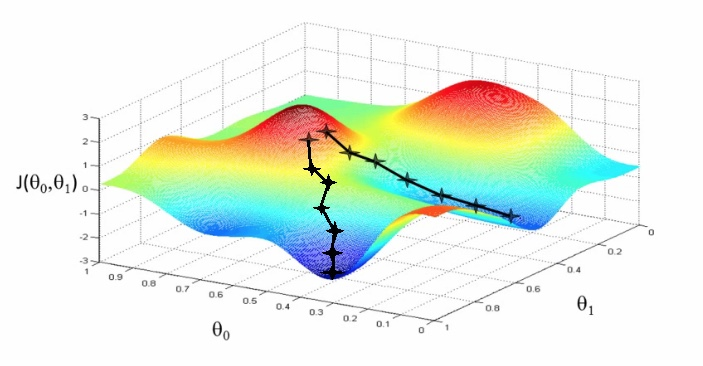### 4.1 具体的计算过程

$$\theta_j := \theta_j - \alpha \frac\partial{\partial\theta_j}J(\theta_0, \theta_1)$$
(for j = 0 and j = 1)

### 4.2 Notation

$\alpha$

• Is a number called the learning rate
• Controls how big a step you take
• If α is big have an aggressive gradient descent
• If α is small take tiny steps
• Too small
• Take baby steps
• Takes too long
• Too large
• Can overshoot the minimum and fail to converge

### 4.3 Computer

$$temp0:= \theta_0 - \alpha \frac\partial{\partial\theta_0}J(\theta_0, \theta_1)$$
$$temp1:= \theta_1 - \alpha \frac\partial{\partial\theta_1}J(\theta_0, \theta_1)$$
$$\theta_0 := temp0$$
$$\theta_1 := temp1$$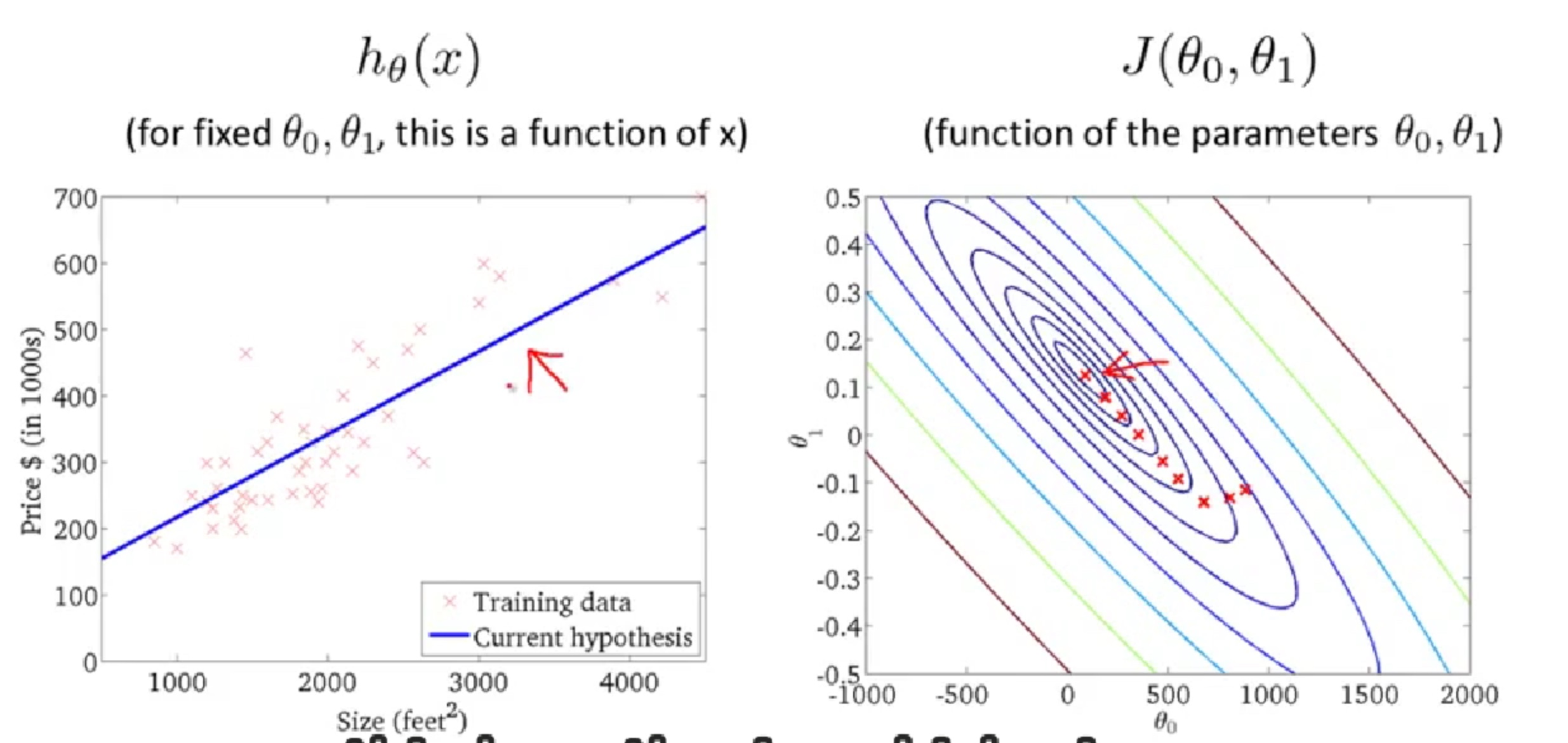#### 4.4 利用梯度下降法求解线性回归问题

$$\frac\partial{\partial\theta_j}J(\theta_0, \theta_1)$$

$$=\frac\partial{\partial\theta_j} * \frac1{2m}\sum_{i=1}^{m}{(h_{\theta}(x^{(i)}) - y^{(i)})^2}$$

$$=\frac\partial{\partial\theta_j} * \frac1{2m}\sum_{i=1}^{m}{(\theta_0 +\theta_1x^{(i)} - y^{(i)})^2}$$

j = 0:
$$\frac\partial{\partial\theta_0}J(\theta_0, \theta_1) = \frac1{m}\sum_{i=1}^{m}(h_{\theta}(x^{(i)}) - y^{(i)})$$
j = 1:
$$\frac\partial{\partial\theta_1}J(\theta_0, \theta_1) = \frac1{m}\sum_{i=1}^{m}(h_{\theta}(x^{(i)}) - y^{(i)})*x^{(i)}$$

### 4.5 梯度下降法的证明

1、如果优化函数存在解析解。例如我们求最值一般是对优化函数求导，找到导数为0的点。如果代价函数能简单求导，并且求导后为0的式子存在解析解，那么我们就可以直接得到最优的参数。

2、如果式子很难求导，例如函数里面存在隐含的变量或者变量相互间存在耦合，互相依赖的情况。或者求导后式子得不到解释解，或者未知参数的个数大于方程组的个数等。这时候使用迭代算法来一步一步找到最优解。

• 当目标函数是凸函数时，梯度下降法的解是全局最优解
• 一般情况下，其解不保证是全局最优解

#### 凸函数

$$f(\frac{x_1+x_2}{2}) \le \frac{f(x_1)+f(x_2)}{2}$$

$$f(px_1+(1-p)x_2) \le pf(x_1)+(1-p)f(x_2)$$

# 要解决的问题

json 反序列化 bean 时，当某个字段在 json 中为 null 时，使用 bean 中声明的默认值。

Person 类我们改造下：

# 解决方案

## 2. JsonCreator 可行吗？

debug 过程：

com.fasterxml.jackson.databind.deser.SettableBeanProperty#deserialize，这个函数的实现是：

Person 类改为：

Person 类如下：

json:

# 解决过程

## 2. debug json 反序列化过程，找到原因

json 反序列化是从

vanillaDeserialize 为 false，最后走到了第 11 行，最后到了

## 4. 根本原因

(补充自 liwei)
jackson 调用了全参构造函数的原因在于@AllArgsConstructor 的构造函数有ConstructorProperties ,jackson在选择构造函数的时候会调用BasicDeserializerFactory._addDeserialzerContructors方法，他首先选择无参构造函数，并遍历所有的构造函数，如果存在具有@ConstructProperties注解的构造函数，则把该构造函数作为默认创建bean的构造函数，如下：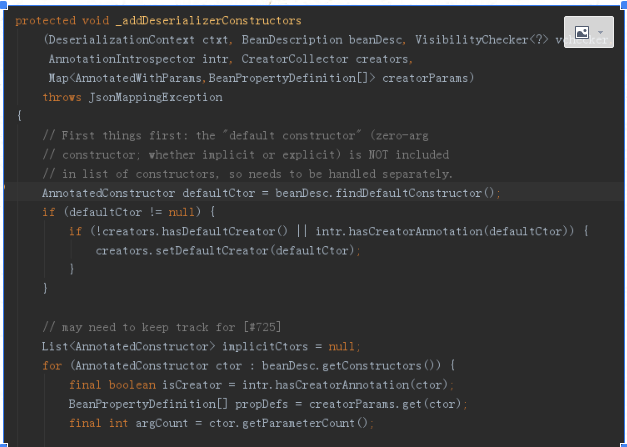# 结论

Lombok 的 @AllArgsConstructor 注解导致 Jackson 反序列化时调用了全参构造函数，将没有出现的字段都赋值为 null 了。

1. 不使用 @AllArgsConstructor
2. 使用 @AllArgsConstructor 但是不让其在全参构造函数上加入 ConstructorProperties 注解，声明方式改为 @AllArgsConstructor(suppressConstructorProperties = true)